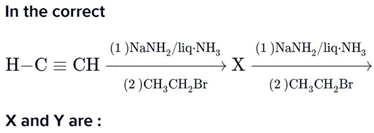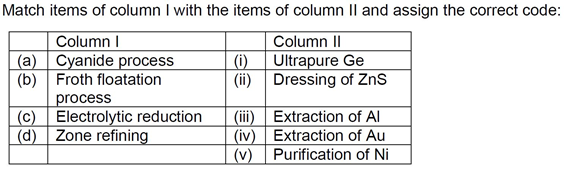# NEET (UG) – 2016 Chemistry Previous Year Paper

46. Consider the molecules CH4,NH3 and H2O. Which of the given statements is false?

(1)The H – C – H bond angle in CH4, the H – N – H bond angle in NH3, and the H – O – H bond angle inH2O are all greater than 90°.

(2)The H – O – H bond angle in H2O is larger than the H – C – H bond angle in CH4.

(3)The H – O – H bond angle in H2O is smaller than the H – N – H bond angle in NH3.

(4)The H – C – H bond angle in CH4 is larger than the H – N – H bond angle in NH3.

Chemistry Notes

Physics Notes

Biology Notes

47.(1) X = 1 – Butyne ; Y = 3 – Hexyne

(2) X = 2 – Butyne ; Y = 3 – Hexyne

(3) X = 2 – Butyne ; Y = 2 – Hexyne

(4) X = 1 – Butyne ; Y = 2 – Hexyne

48. Among the following, the correct order of acidity is:

(1) HClO3 < HClO4 < HClO2 < HClO

(2) HClO < HClO2 < HClO3 < HClO4

(3) HClO2 < HClO < HClO3 < HClO4

(4) HClO4 < HClO2 < HClO < HClO3

49. The rate of a first-order reaction is 0.04 mol l−1s−1 at 10 second and 0.03 mol l−1s−1 at 20 seconds afterinitiation of the reaction. The half-life period of the reaction is:

(1)24.1 s                                              (2)34.1 s

(3)44.1 s                                              (4)54.1 s

Chemistry Notes

Physics Notes

Biology Notes

50. Which one of the following characteristics is associated with adsorption?

(1)ΔG is negative but ΔH and ΔS are positive

(2)ΔG,ΔH and ΔS all are negative

(3)ΔG and ΔH are negative but ΔS is positive

(4)ΔG and ΔS are negative but ΔH is positive

51. In which of the following options the order of arrangement does not agree with the variation of propertyindicated against it?

(1)Al3+ < Mg2+ < Na+ < F (increasing ionic size)

(2)B < C < N < O (increasing first ionization enthalpy)

(3)I < Br < Cl < F (increasing electron gain enthalpy)

(4)Li < Na < K < Rb (increasing metallic radius)

52. Which of the following statements is false?

(1)Mg2+ ions form a complex with ATP.

(2)Ca2+ ions are important in blood clotting.

(3)Ca2+ ions are not important in maintaining the regular beating of the heart.

(4)Mg2+ ions are important in the green parts of plants.

53. Which of the following statements about hydrogen is incorrect?

(1) Hydrogen has three isotopes of which tritium is the most common.

(2) Hydrogen never acts as cation in ionic salts.

(3) Hydronium ion, H3O+ exists freely in solution.

(4) Dihydrogen does not act as a reducing agent.

Chemistry Notes

Physics Notes

Biology Notes

54. The correct statement regarding a carbonyl compound with a hydrogen atom on its alpha carbon, is:

(1) A carbonyl compound with a hydrogen atom on its alpha-carbon never equilibrates with its correspondingenol.

(2) A carbonyl compound with a hydrogen atom on its alpha-carbon rapidly equilibrates with its correspondingenol and this process is known as aldehyde-ketone equilibration.

(3) A carbonyl compound with a hydrogen atom on its alpha-carbon rapidly equilibrates with its correspondingenol and this process is known as carbonylation.

(4) A carbonyl compound with a hydrogen atom on its alpha-carbon rapidly equilibrates with its correspondingenol and this process is known as keto-enol tautomerism.

55. MY and NY3, two nearly insoluble salts, have the same Ksp values of 6.2×10−13 at room temperature. Whichstatement would be true in regard to MY and NY3?

(1) The molar solubilities of MY and NY3 in water are identical.

(2) The molar solubility of MY in water is less than that of NY3.

(3) The salts MY and NY3 are more soluble in 0.5 M KY than in pure water.

(4) The addition of the salt of KY to solution of MY and NY3 will have no effect on their solubilities.

57. Nutural rubber has:

(1) All cis – configuration

(2) All trans – configuration

(3) Alternate cis – and trans – configuration

(4) Random cis – and trans – configuration

Chemistry Notes

Physics Notes

Biology Notes

58.(1) (a) – (iv)           (b)- (ii)       (c) – (iii)       (d) – (i)

(2) (a) – (ii)            (b)- (iii)      (c) – (i)         (d) – (v)

(3) (a) – (i)             (b)- (ii)       (c) – (iii)       (d) – (iv)

(4) (a) – (iii)           (b)- (iv)      (c) – (v)        (d) – (i)

59. Which one of the following statements is correct when SO2 is passed through acidified K2Cr2O7 solution?

(1) The solution turns blue.

(2) The solution is decolourized.

(3) SO2 is reduced.

(4) Green Cr2(SO4)3 is formed.

60. The electronic configurations of Eu (Atomic no. 63), Gd (Atomic NO. 64) and Tb (Atomic No. 65) are:

(1) [Xe]4f76s2 ,[Xe]4f86s2 and [Xe]4f85d16s2

(2) [Xe]4f65d16s2 ,[Xe]4f75d16s2 and [Xe]4f96s2

(3) [Xe]4f65d16s2 ,[Xe]4f75d16s2 and [Xe]4f85d16s2

(4) [Xe]4f76s2 ,[Xe]4f75d16s2 and [Xe]4f96s2

61. Two electrons occupying the same orbital are distinguished by:

(1) Principal quantum number

(2) Magnetic quantum number

(3) Azimuthal quantum number

(4) Spin quantum number

62. When copper is heated with conc. HNO3 it produces:

(1) Cu(NO3)2 and NO2

(2) Cu(NO3)2 and NO

(3) Cu(NO3)2,NO and NO2

(4) Cu(NO3)2 and N2O

63. Which of the following reagents would distinguish cis-cyclopenta-1, 2-diol from the trans-isomer?

(1) Acetone                                 (2) Ozone

(3) MnO2                                    (4) Aluminium isopropoxide

64. The correct thermodynamic conditions for the spontaneous reaction at all temperatures is:

(1) ΔH < 0 and ΔS = 0

(2) ΔH > 0 and ΔS < 0

(3) ΔH < 0 and ΔS > 0

(4) ΔH < 0 and ΔS < 0

65. Lithium has a bcc structure. Its density is 530 kg m−3 and its atomic mass is 6.94 g mol−1. Calculated the edgelength of a unit cell of Lithium metal. (NA=6.02×1023 mol−1)

(1) 154 pm                       (2) 352 pm

(3) 527 pm                       (4) 264 pm

66. Which one of the following orders is correct for the bond dissociation enthalpy of halogen molecules?

(1) I2 > Br2 > Cl2 > F2

(2) Cl2 > Br2 > F2 > I2

(3) Br2 > I2 > F2 > Cl2

(4) F2 > Cl2 > Br2 > I2

67. Which of the following is an analgesic?

(1) Novalgin

(2) Penicillin

(3) Streptomycin

(4) Chloromycetin

68. Equal moles of hydrogen and oxygen gases are placed in a container with a pin-hole through which both canescape. What fraction of the oxygen escape in the time required for one-half of the hydrogen to escape?

(1) 1 / 8

(2) 1 / 4

(3) 3 / 8

(4) 1 / 2

69. Consider the nitration of benzene using mixed conc. H2SO4 and HNO3. If a large amount of KHSO4 is added tothe mixture, the rate of nitration will be:

(1) Faster

(2) Slower

(3) Unchanged

(4) Doubled

70. Predict the correct order among the following:

(1) Lone pair – lone pair > lone pair – bond pair > bond pair – bond pair

(2) Lone pair – lone pair > bond pair – bond pair > lone pair – bond pair

(3) Bond pair – bond pair > lone pair – bond pair > lone pair – lone pair

(4) Lone pair – bond pair > bond pair – bond pair > lone pair – lone pair